##### Physics I: 501 Practice Problems For Dummies (+ Free Online Practice)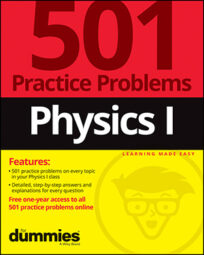Using physics, you can show that when two objects collide, their total momentum is conserved. For example, you can calculate how two objects colliding will result in an increase in total mass but a decrease in velocity.

Here are some practice questions that you can try.

## Practice questions

1. What quantity is always conserved during elastic collisions but never during inelastic ones?

2. What quantity is always conserved during a collision?

3. An astronaut does an experiment on the International Space Station in which a glob of polymer with velocity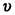runs into a second, stationary, equal-massed glob of polymer. If the two globs stick together after the collision, what is the velocity of the combined glob in terms of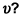The following are the answers to the practice questions:

1. kinetic energy

Although momentum is always conserved during a collision — whether elastic or inelastic — kinetic energy isn't conserved during inelastic collisions.

2. momentum

During a collision of two objects, each object exerts a force upon the other object. Newton's third law says those forces are equal in magnitude and opposite in direction. Because an equal force is exerted for an equal time on each object, the magnitude of the impulse is the same for each object. The impulse for each object is in an opposite direction, so the total impulse is zero. But impulse is just the change in momentum, so the change in momentum is zero. Momentum is always conserved during a collision.

3.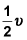Initially, the stationary glob has no momentum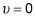so all the momentum in the "system" — composed of the two globs — is maintained by the moving glob. After the collision, that momentum now supports two globs — twice the mass it was originally propelling. Because momentum is the product of mass and velocity, the only way to keep the momentum constant is for the mass to be multiplied by a factor of 2 at the same time the velocity is divided by a factor of 2.